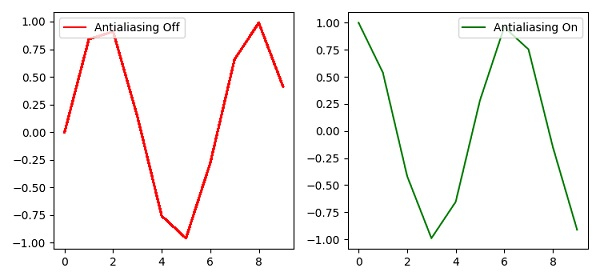# Plot curves to differentiate antialiasing in Matplotlib

To differentiate antialiasing through curves, we can take the following Steps −

• Add a subplot to the current figure, using the subplot() method, where nrows=1, ncols=2 and index=1.

• Plot the curve using the plot() method, where antialiased flag is false and color is red.

• Place the legend at the upper-left corner using the legend() method.

• Add a subplot to the current figure, using the subplot() method, where nrows=1, ncols=2 and index=2.

• Plot the curve using the plot() method, where antialiased flag is true and color is green.

• Place the legend at the upper-right corner using the legend() method.

• To display the figure, use the show() method.

## Example

import numpy as np
from matplotlib import pyplot as plt
plt.rcParams["figure.figsize"] = [7.50, 3.50]
plt.rcParams["figure.autolayout"] = True
plt.subplot(1, 2, 1)
plt.plot(range(10), np.sin(range(10)), antialiased=False, label='Antialiasing Off', c="red")
plt.legend(loc="upper left")
plt.subplot(1, 2, 2)
plt.plot(range(10), np.cos(range(10)), antialiased=True, label='Antialiasing On', c="green")
plt.legend(loc="upper right")
plt.show()

## Output